Exercises

# Subtraction 3

Subtraction fluency within 20 is the focus of this guided lesson for second graders. Kids will be tasked with mental subtraction, as well as with using manipulatives and drawings to add and subtract larger numbers. Word problems will help to round out the lesson, as kids follow the step-by-step method of solving subtraction word problems. For even more practice in this skill, check out our subtraction worksheets.
This lesson includes 5 printable learning activities.

## The Shark DentistAs sharks, Dr. Chompers' patients are great at biting, but aren't so great at counting. Help Dr. Chompers take care of what teeth his patients have left in this story that practices subtraction.

## Secret Agent Addition and Subtraction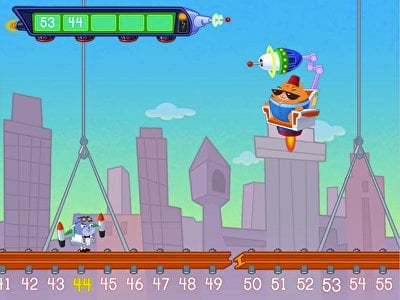Help Agent 00K9 dodge the evil El Gato in this fun-filled, spy-themed number line game! Kids must quickly add or subtract one-digit and two-digit numbers to reveal the secret code and save the day. This interactive math game encourages students to use a number line to strengthen mental math skills.

## Ski Race: Subtraction Within 20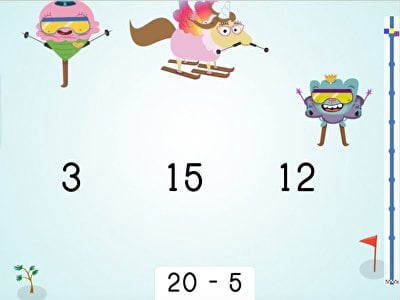Kids glide into subtraction in this silly math game. Young learners practice subtraction within 20, building mental math fluency with every correct answer. Kids will love cruising down the mountain in this fast-paced game, and educators will love how tackling simple subtraction problems will help children build important math skills.

## Match: Subtraction Facts within 20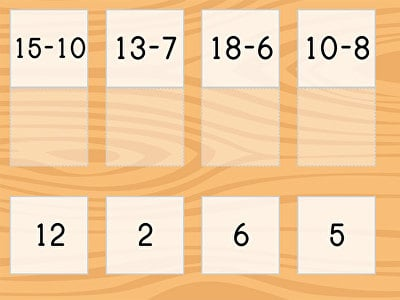Match up these subtraction facts! What is 4 less than 10? What is 19 minus 9? In Match: Subtraction Facts Within 20, students practice reading and identifying common subtraction equations with numbers 0-10 and numbers 11-20. Second graders who are learning about basic subtraction within 20 can use this online game to practice their arithmetic at home or in the classroom.

## Ski Racer: Subtraction Within 30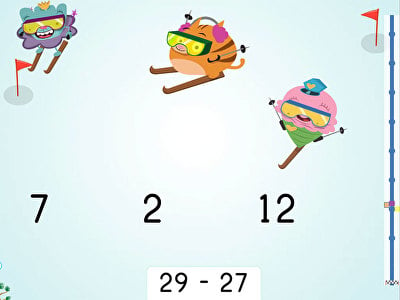Ski racers practice subtraction within 30 in this fun, fast-paced math game designed for second graders! Players choose a Brainzy avatar and then race down the snowy mountain, solving two-digit subtraction problems as quickly as they can in order to beat the other racers across the finish line. The quicker they solve the problems, the more likely they are to win the race!

## Ski Racer: Three-Digit Subtraction and Finding 10 Less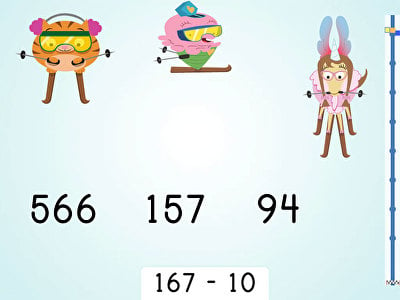Help your child subtract 10 from three-digit numbers with confidence with this online game that is as entertaining as it is educational. In Ski Racer: Three-Digit Subtraction and Finding 10 Less, young learners use their mental math skills to ski down the mountain while solving three-digit subtraction problems quickly and correctly. This resource is a great way to help second graders hone their math and pattern recognition skills.

## Add and Subtract Within 1000Time for a shopping run! Kids practice addition and subtraction with manipulatives in this silly shopping game. Children choose 1, 10, or 100 packs of toilet paper to create three-digit numbers shown onscreen. By connecting abstract numbers with concrete manipulatives, kids build skills that will help them master math fluency.

## Two-Step Subtraction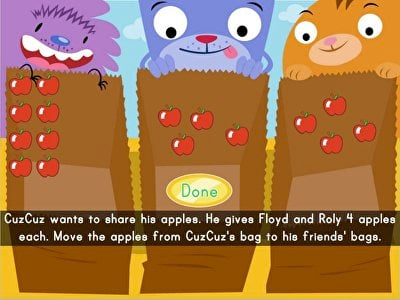The gang is going apple picking, and they need help getting just the right amount of bushels. Kids help the group collect just the right number by subtracting apples in two-step equations. Using manipulatives helps kids strengthen essential math skills to help prepare them for mental math equations.